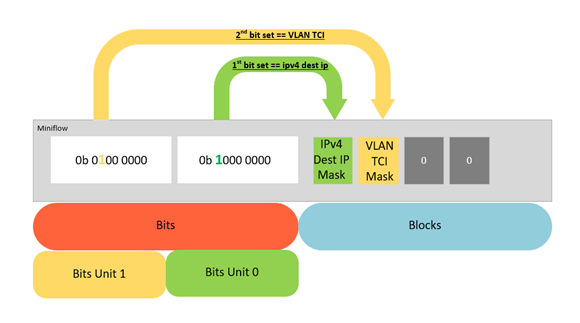# Open vSwitch subtable-show Tool

ID 659943
Updated 3/24/2020
Version Latest
PublicBy

## Overview

This article describes the subtable-show tool in Open vSwitch, available as of OvS 2.13. It will focus on optimizations applied to the datapath classifier (known as DPCLS) in OvS 2.12. In this article we explain how miniflows work, how the datapath classifier iterates over them, and how compile time constants can provide optimized lookup functions.

## Miniflow

A miniflow is a data structure in OvS which stores metadata. Both packets and subtables have miniflows. The subtable-dump tool only focuses on accessing the subtable miniflow. The core aspects of a miniflow are depicted in the Figure 1.Figure 1: OvS MiniFlow Overview

On the left-hand side of the miniflow there are bits consisting of unit 0 and unit 1 and on the right-hand side there are the blocks of actual data. The bits that are set in the bitmask on the left-hand side tell us what values are in the blocks on the right-hand side. In Figure 1, there are bits set for an IPv4 destination address and a VLAN TCI, and as shown in the diagram there are corresponding bits set for these block values.

## Optimization of the DPCLS

In OvS 2.12 the datapath classifier was refactored and is now a generic scalar function. In addition, subtables now have specialised miniflow lookup functions. In the scalar function, dpcls_subtable_lookup_mf_u0wX_u1wY the value X is the quantity of bits set (popcount) in unit 0, while Y is the quantity of bits set in unit 1, as shown in Figure 2 below.Figure 2: Miniflow Representation

The advantage of knowing the X and Y bit counts at compile time is that the compiler can statically resolve loop trip counts. This is in contrast to previous versions of OvS which had to be capable of looping over any number of bits, as it was not possible for the compiler to statically determine the loop trip count. Currently the DPCLS has implemented 3 specialised functions with different bit widths. However, if the subtable has a different quantity of bits set, then the application doesn’t benefit from the gain and will default to using the dpcls_subtable_lookup_generic() function, as shown in Figure 3.Figure 3: DPCLS functions in OvS 2.12

With the new refactor of the DPCLS in OvS 2.12, it is easy to add more specialised lookup functions. However, it is difficult to know what values X and Y should be set to. A solution to this is to utilise the ovs-appctl dpctl/dump-flows command which is available as of OvS 2.13:

dump-flows -m

This command prints to the console all flow entries in the datapath (dp) flow table. With the options “-m” or “--more”, the output includes all wildcarded fields, as well as a new field called dp-extra-info. A sample output is shown in Figure 4.Figure 4: Console Output of dump-flows -m

The dp-extra-info field contains the miniflow bits for each subtable installed in the datapath. The above example shows a subtable matching against the following flow:

ovs-ofctl add-flow br0 dl_type=0x0800,nw_src=21.2.10.1/24,actions=output:2

i.e. it matches on an IPv4 source address of 21.2.10.1/24.

These miniflow bit values (4,2) can then be used for an optimised version of the lookup function for this particular subtable. In the optimized version, the compiler is able to determine loop trip counts at compile time, and perform loop unrolling as an optimization. This has a significant impact on the runtime performance of the code. If these values are not present at compile time, the generic implementation of this lookup function will be used. Note that the generic version of the function will be able to search any subtable bit count (x,y), so it is more flexible but also less performant than the specialized lookup functions.

The table below shows examples of flows which will result in specialised subtable miniflows:

 Flow Subtable miniflow ovs-ofctl add-flow br0 udp,tp_src=1234,actions=output:2 4,1 ovs-ofctl add-flow br0 dl_vlan=1,udp,tp_src=1234,actions=output:2 5,1

## Conclusion

In this article we looked at the core aspects of the miniflow data structure in OvS. We also described the addition of generic scalar functions to the DPCLS. Finally, we explained how to extract subtable miniflow bits in OvS 2.13 and its importance in terms of performance gains.